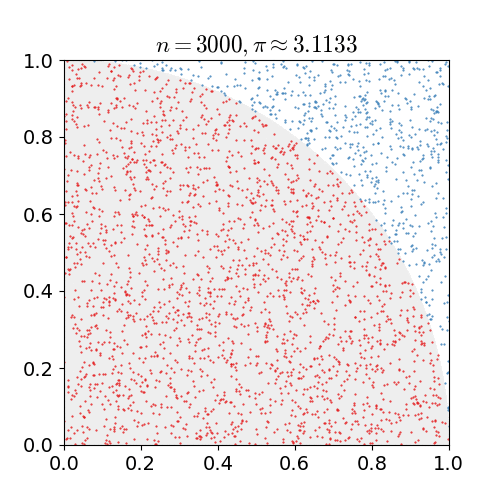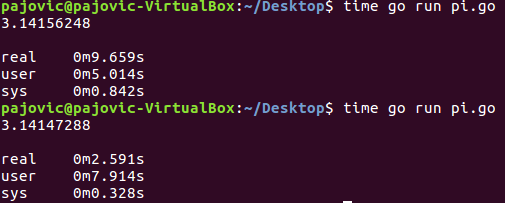# Go Language Competitiveness Part IIThe first part describes the basic concepts of Go competitiveness. In this section, we will explore more advanced concepts of competitiveness that govern cross-thread synchronization. Depending on the problems they are solving, it may often be necessary to access more or more on-call resources. In such situations, problems such as race conditions, mutual blockages or starvation can arise. As we reduce the chances of problems, the Go programming language introduces a novelty that allows threads to share resources, using a “channel”.

### Channels

Channels, as the word implies, allow data to be exchanged between threads using the <-

ch <- value // pass the value to the ch channel
v: = <- ch // get the data from the channel and assign a value to the variable

Logically, data exchange takes place in the direction of the arrow. In order to use a channel at all, we need to create it using the make keyword and indicate the type of data to be exchanged.

ch: = make (chan int)

The party receiving the information waits until the information is sent. This principle allows for synchronization between threads without traffic lights or conditioning variables, as was done in a programming language such as C.

package main

import “fmt”

func sum (s [] int, c chan int) {
sum: = 0
for _, v: = range s {
sum + = v
}
c <- sum // send sum to channel c
}

func main () {
s: = [] int {7, 2, 8, -9, 4, 0}

c: = make (chan int)
go sum (s [: len (s) / 2], c)
go sum (s [len (s) / 2:], c)
x, y: = <-c, <-c // we get the results of functions through channel c

fmt.Println (x, y, x + y)
}

The program execution result is -5 17 12.

In the previous example, we saw how a budget can be split into multiple threads, and only after the completion of their individual calculations is the final result calculated. Analogous to the previous example, we can write a program that approximates the value of the number π using the Monte Carlo method.The Monte Carlo method works according to the sampling principle. To solve the approximate determination of the number π we will use the square and the circle inscribed in it. If the radius of the circle is r, the side of the square is 2r, so the area of the square is 4r2, while the area of the circle is squared r2π. As we do not know the surfaces, we will have to approximate them by a random selection of points. After n iterations, the ratio of the total number of points and the number of points in the circle will be equal to the approximate ratio of the surfaces, from which we can calculate the value of π.

package main

import (
“fmt”
“time”
“math / rand”
)

const n = 1000000 // number of samples per thread
const t = 16 // number of threads

func work (c chan float64) {// function that counts the number π
s: = rand.NewSource (time.Now (). UnixNano ())
r: = rand.New (s)
cn: = 0
for i: = 0; i <n; i ++ {
x: = r.Float64 ()
y: = r.Float64 ()

if x * x + y * y <= 1 {
cn ++
}
}
c <- float64 (4) * float64 (cn) / float64 (n)
}

func main () {
c: = make (chan float64)
var sum float64 = 0
for i: = 0; i <t; i ++ {
go work (c) // start threads
}
for i: = 0; i <t; i ++ {
sum + = <- c // summarize the thread results
}
fmt.Println (sum / t) // final bill
}

The result of executing the program is as follows

3.14159275

In this example, we can see how, if we have more processor cores available, we can distribute the work to multiple threads and get them up to speed, or increase the number of samples and get them accurate at the same time. Now we will perform testing where in the first example we will assign the whole job to one thread, while in the second example we will distribute the total number of samples evenly over the four threads. The test result is as follows:Real represents the actual execution time, from program start to finish. This includes the processor time used by other processes and the time spent by the process in blocking. On the other hand, the user represents only the processor time that the process used to execute. If process execution is split into multiple threads, the user represents the total execution time of all threads on all processing units. From the attached example we can see that by dividing the process into four threads, we have obtained an acceleration of almost four times!

### Buffer channels

If there is a need to send more data through a channel, we can use a buffer channel. All we have to do is indicate the size of the buffer when creating the channel.

ch: = make (chan int, 100)

When using a buffer channel, care must be taken not to overfill the buffer.

package main

import “fmt”

func main () {
ch: = make (chan int, 2)
ch <- 1
ch <- 2
// ch <- 3 if we comment on the line we will get an error because we have overflowed the buffer
fmt.Println (<- ch, <-ch)
}

The result of the execution of this program is 1 2.

### Select command

Select command allows the thread to listen on multiple channels. The program is blocked until one of the channels is ready, after which data is downloaded from the ready channel. If more than one channel is ready, the channel is selected by random selection.

package main

import (
“fmt”
“time”
)

func main () {
c1: = make (chan string) // create two channels
c2: = make (chan string) // through which string type data will be transmitted

go fun1 (c1) // start

select {// select command that listens on two channels
case s1: = <- c1: // case that the data first comes from channel c1
fmt.Println (s1)
case s2: = <- c2: // case that the data first comes from channel c2
fmt.Println (s2)
}
}

func fun1 (ch chan string) {
time.Sleep (2 * time.Second)
ch <- “first function”
}

func fun2 (ch chan string) {
time.Sleep (1 * time.Second)
ch <- “second function”
}

The result of executing the program will be a second function, after which the program ends.

Although channels are an excellent form of communication between threads, they should not be used at all costs. What if we didn’t need communication at all? What if we wanted to make sure that only one thread could access a variable at a given time? When developing competitive programs, it is very important to ensure such situations are avoided in order to avoid a race situation. A race condition can occur if multiple threads access the same critical section at a time. The principle of mutual exclusion allows us to prevent the condition of the race. The simplest structure that allows us to mutually exclude is a mutex (short for mutual exclusion), a binary semaphore, which can be unlocked or locked. The sync package provides us with the Mutex structure with its two methods, Lock and Unlock.

### The Philosopher’s Problem at Dinner

One of the most well-known problems of competitive programming is certainly the problem of philosophers at dinner. It was first exhibited by Dijkstra in 1965. This problem is important because it reflects the basic problems of mutual blocking and starvation. Five philosophers live in a house where a table is set for them. Every philosopher’s life comes down to thinking and eating. All philosophers agreed that spaghetti was the only food that helped them think. Due to a lack of skill, every philosopher needs two forks to eat spaghetti. It is necessary to simulate a ritual that will allow philosophers to eat. The algorithm must satisfy mutual exclusion (two philosophers cannot use the same fork at the same time) and mutual blocking and starvation must be avoided. Mutual blocking can occur, for example, when each philosopher takes a fork from his left. When it reaches the right, the so-called a circular wait and a blockage occurs. One possible solution:

package main

import (
“fmt”
“sync”
“time”
“math / rand”
)

const n = 5 // number of philosophers
var mutex sync.Mutex
var wg sync.WaitGroup
var sticks [n] int // synchronization helper string

func getLeftIndex (i int) int {// function that returns the index of the left fork from the helper string
if i == 0 {
return n – 1
} else {
return i – 1
}
}

func getRightIndex (and int) int {// function that returns the index of the right fork from the helper string
if i == n – 1 {
return 0
} else {
return i + 1
}
}

func doWork (and int) {
id: = i

for {
fmt.Printf (“Philosopher% d thinks \ n”, id)
time.Sleep (2 * time.Second)

for {
mutex.Lock () // we lock the mutex to provide a critical section

if sticks [id] == 2 {// if we have forks available
sticks [getLeftIndex (id)] – // reduce their number
sticks [getRightIndex (id)] – // to prevent others from doing so

mutex.Unlock () // unlock the mutex and leave the critical section

fmt.Printf (“Philosopher% d eats \ n”, id)
time.Sleep (1 * time.Second)

mutex.Lock () // we lock the mutex to provide a critical section

sticks [getLeftIndex (id)] ++ // magnify fork condition
sticks [getRightIndex (id)] ++ // for other philosophers to access

mutex.Unlock () // unlock the mutex and leave the critical section
break
} else {
mutex.Unlock () // if we didn’t have forks we unlock the mutex
time.Sleep (time.Duration (rand.Intn (n)) * time.Second) // and pause for a specific time
}
}
}
}

func main () {

for i: = 0; i <n; i ++ {
sticks [i] = 2 // we set the initial values ​​of the auxiliary string
}
for i: = 0; i <n; i ++ {
go doWork (i) // start the threads
}
wg.Wait ()
}

An example of running a simulation is:

Philosopher 4 thoughts
Philosopher 0 thoughts
Philosopher 1 thinks
Philosopher 2 thinks
Philosopher 3 thoughts
Philosopher 0 eats
Philosopher 3 eats
Philosopher 3 thoughts
Philosopher 0 thoughts
Philosopher 4 eats
Philosopher 1 eats
Philosopher 4 thoughts
Philosopher 1 thinks
Philosopher 3 eats
Philosopher 0 eats

In the previous examples, we have seen how we can develop competitive programs in the Go language. Of course, program writing and application development take a much broader picture. The Go programming language is not perfect. One major drawback is the lack of support for generic programming. Also, the concept of object-oriented programming is somewhat different from some traditional languages such as Java, C # or C ++. As a relatively young language, a large number of libraries have not yet been developed to support developers in various fields of application.

### Conclusion

We have seen that Google’s team paid particular attention to competitiveness while developing the Go programming language. In this article, you were introduced to the basic principles of Go competitiveness. We have seen that writing competing programs in Go is very simple and has certain features that other languages or libraries do not have. The next time you develop an application that requires competitive execution, consider using the Go language.

Likes:
9 0
Views:
1344
Article Categories:
PROGRAMMINGTECHNOLOGY